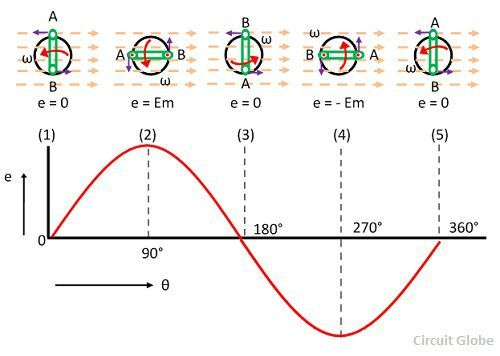# Generation of Alternating Voltage

The voltage which changes polarity at regular interval of time is known as the alternating voltage. The one complete cycle of an alternating quantity consists two half cycles. And the direction of a half cycle changes after every particular interval of time. The machine which generates the alternating voltage is known as the alternator.

The alternating voltage is generated in two ways.

• By rotating the coil inside the uniform magnetic field at constant speed
• By rotating the magnetic field around the stationary coil at the constant speed.

In small AC generators, the coil rotates between the magnetic field, whereas in large ac generator the magnetic field rotates around the coil because of some economical consideration.

### Process of Generating Alternating Voltage

Consider the stationary coil places inside the uniform magnetic field. The load is connected across the coil with the help of brushes and the slip rings. When the coil rotates in the anticlockwise direction at constant angular velocity ω the electromotive force induces in the coil. The cross-sectional view of the coil at the different position is shown in the figure below.

The magnitude of the emf induced in the coil depends on the rate of the flux cut by the conductor. The figure below shows that the no current induces in the coil when they are parallel to the magnetic line of forces. i.e., at the position (1), (2) and (3). And the total flux cut by the conductor becomes zero.The magnitude of the induces emf becomes maximum when the conductor becomes perpendicular to the magnetic line of force. The conductor cuts the maximum flux at this position.

The direction of the emf induces in the conductor is determined by Fleming’s right-hand rule. When the coil is at position (2) the emf induces in the outward direction of the conductor whereas at position (4) the direction of the inducing emf becomes inward.

In other words, the direction of emf induces in the conductor at position (2) and (4) becomes opposite to each other.